ArcGIS has numerous tools to calculate topographic elements of all kinds, including volumetric calculations. In this post, we will learn how to calculate the volume of a polygon.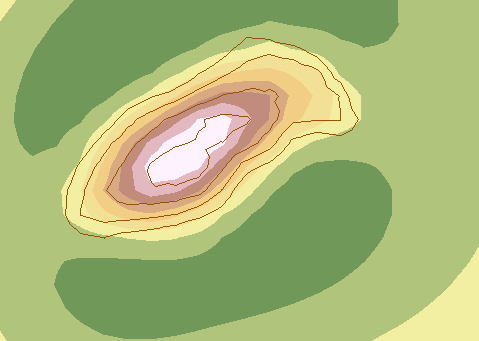Let’s start with this main shape, a series of contour lines extracted from a raster. They represent at which height stands each one and this is how we will study the existing volume of a mountain.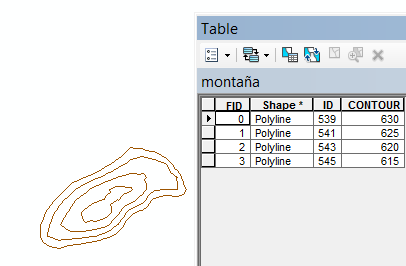First, let’s create the raster shape.

Use the “Topo to raster” tool, located in: 3D Analyst Tools > Raster Interpolation> Topo to Raster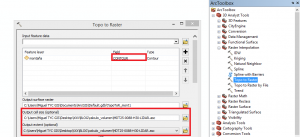We have to realize that in the option “field” it will be selected the field that refers to height, in this case the “contour” field.

The cell size and extent field are selected form the DTM where we have obtained the contour lines.

We will obtain something similar to this:We now need to delineate the calculation area.

We will extract by mask and for that we need to have a mask that will be the mountain´s base.

We will select the base line through the specified tool and choose “extract” right clicking the layer.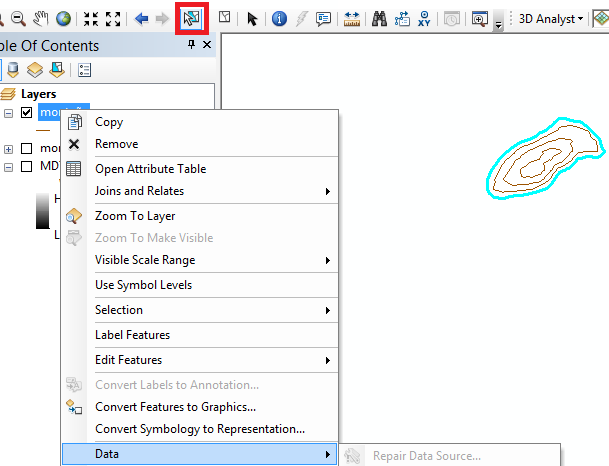As this is a line, we need to convert it to polygon: Data Management Tools > Features > Feature to PolygonTherefore we have the base polygon and we will extract by mask using it as the mask: Spatial Analyst Tools > Extraction > Extract by Mask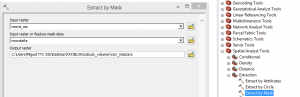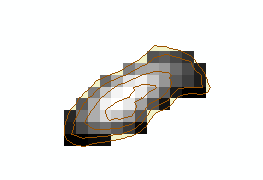Before calculating the volume, we need to know if we will decide the option “Above” or “Below” and also have the Environment settings prepared regarding the coordinates and raster analysis.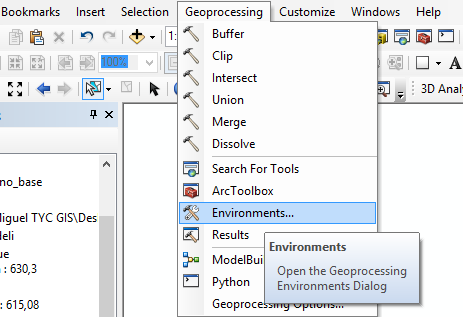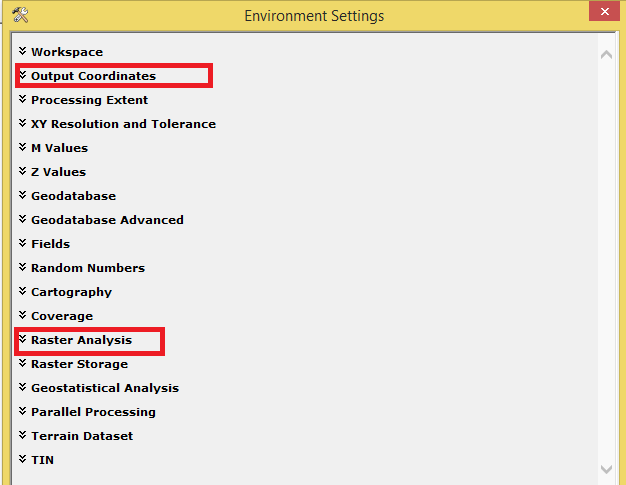As in this case we want to calculate the volume inside a mountain, we will select the option “Above”. If the volume would be the one of a lake, the option should be “Below”.

3D Analyst Tools > Functional surface > Surface Volume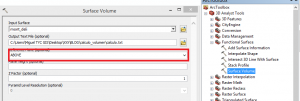We have to open the generated file in .txt format in the folder where it has been saved.This document indicates that the 2D area is 23125 m2, the 3D area is 23450 m2 and the volume is 196067m3.

Quality training taught by professionals

RECOMMENDED COURSE